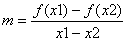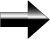Differentiation

Differentiation is one of the two basic concepts of calculus.  Differentiation tells us about how something is changing.  When we differentiate a function, we are taking its derivative.

This lesson will show you the basic concept of the derivative.  Remember, a derivative represents the rate of change of a function.

We approximate this curve by drawing a straight green line between two points on the graph.  To find the slope of this line, we use the familiar definition of slope:If we say that x2 = x1 + Δx, our slope then becomes:We can see that the closer x1 and x2 get to each other, the shorter our straight line and the more closely the straight line resembles the curve.  Therefore, we take the limit of our difference quotient as Δx approaches zero.  Doing this gives us the definition of the derivative:Main Calculus Page